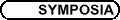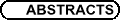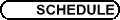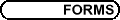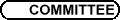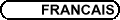Dynamical Systems / Systèmes dynamiques
(Org: Michael A. Radin, Rochester Institute of Technology)

BERNARD BROOKS, Rochester Institute of Technology
Rumour Propagation Modeled as a Dynamical System on a Network

Mathematical models of rumour propagation have traditionally used a `rumour as epidemic' approach. Such models are loosely based on a reaction-diffusion system and grossly simplify the connections between the individual people in question. In most cases they are thought of as all being infinitely and continuous connected. Instead, we will consider a population connected together in a given network architecture. How does the architecture of the network itself affect propagation? The population will have two subgroups, each with a different set of values such as Liberals and Conservatives. How does the distribution of these subgroups on the network affect rumour propagation?

SHARENE BUNGAY, University of Waterloo, Waterloo, Ontario
Numerical analysis of a bi-directional ring neural network with delayed feedback

Many physiological systems involve rings of similar neurons. Such neural networks can be mathematically modelled as a system of delay differential equations, where the coupling between neurons is often modelled as simple step functions or nonlinear sigmoidal functions. Here, we investigate a three-dimensional bi-directional symmetric ring neural network with delayed coupling and self feedback. This model was numerically analyzed to identify and compute the different types of periodic solutions arising from equivariant Hopf bifurcations. This work was performed with the bifurcation package DDE-BIFTOOL which also allowed the computation of the stability of the various periodic solutions. Secondary bifurcations and multistability near codimension two bifurcation points are also investigated. These results are complemented by numerical simulations of the system using XPPAUT.

MONICA COJOCARU, University of Guelph, Guelph, ON
Projected Dynamical Systems and Evolutionary Variational Inequalities with Applications to Dynamic Traffic Networks

In this talk we make explicit the connection between projected dynamical systems on Hilbert spaces and evolutionary variational inequalities. We give a novel formulation that unifies the underlying constraint sets for such inequalities, which arise in time-dependent traffic network and spatial price equilibrium problems. We provide a traffic network numerical example in which we compute the curve of equilibria.

This is joint work with Patrizia Daniele, University of Catania, and Anna Nagurney, Isenberg School of Management, University of Massachusetts.

MARSHALL HAMPTON, University of Minnesota
On Smale's 6th Problem: A solution in the four-body case

In 1998 Smale formulated 18 problems for the 21st century, the sixth of which was: prove that there are finitely many relative equilibria for positive masses in the planar n-body problem. In joint work with Richard Moeckel, we have proven this result for n = 4.

KRIS HEIDLER, University of Guelph, Guelph, Ontario N1G 2W1
Using the Collage Method on the Lorenz System

It is well known that the Lorenz system

 x¢
 = a(y-x)
 y¢
 = rx-y-xz
 z¢
 = xy-bz
generates a "butterfly attractor" for the standard parameter value choice of a=10, b=[ 8/3], and r=28. Given a dataset of observed solution component values, with parameters in this chaotic realm, the inverse problem of approximating the parameter values is considered. In this talk, we investigate how well the collage method handles this problem.

HERBERT KUNZE, University of Guelph
Using Collage Coding to Solve Inverse Problems

Broad classes of inverse problems in differential and integral equations can be viewed as seeking to approximate a target x of a metric space X by fixed points of contraction maps on X. Each contraction map depends on the parameters of the underlying system-for example, chemical reaction rates, population interaction rates, Hooke's constants-and such problems frequently appear in the parameter estimation literature. The "collage method" attempts to solve such inverse problems by finding a map Tc that sends the target as close as possible to itself. In this talk, after briefly introducing the framework which surrounds the collage method, I will discuss some recent applications, as well as some technical issues.

TCHAVDAR MARINOV, Department of Mathematical Sciences, University of Alberta, Edmonton
Solitary-Wave Solutions Identification of Boussinesq and Korteweg-de Vries Equations as an Inverse Problem

A special numerical technique has been developed for identification of solitary wave solutions of Boussinesq and Korteweg-de Vries equations. Stationary localized waves are considered in the frame moving to the right. The original ill-posed problem is transferred into a problem of the unknown coefficient from over-posed boundary data in which the trivial solution is excluded. The Method of Variational Imbedding is used for solving the inverse problem. The generalized sixth order Boussinesq equation is considered for illustration.

MICHAEL A. RADIN, Rochester Institute of Technology
Trichotomy behavior of solutions of a rational difference equation

We will investigate a particular rational difference equation:

 y[n+1] = (py[n-1]+y[n-2])/(q+y[n-2])
and discover how the properties of the given equation and the long term behavior of the positive solutions depends on the relationship between the parameters and not on the initial conditions.

WEIGUANG YAO, York University, Department of Mathematics and Statistics
Immune System Memory Realization in A Lattice Population Model

A general process of the immune system consists of effector stage and memory stage. It is not fully understood how the memory stage forms and how the system switches from the effector stage to the memory stage. Existing mathematical models of the immune system can describe either the effector stage or the memory stage, but not both. We formulate a mathematical model based on a recently developed cellular automata model for influenza A virus, and show that these two stages can be smoothly realized in our model. The numerical simulations of our model agree with the clinical observation very well.